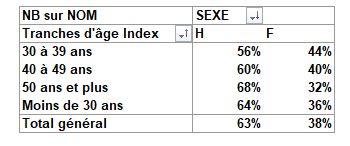# % calculation from a table pivoted or groupedby

Hello there,

New to Knime, I find this tool very great. But, something is annoying me at the time because I can’t succeed in doing what I’d like.

What I’d like is:
I have a tab like this one:And I would like to have the figures in % by lines and by columns so two differents transformations:Impossible to perform it. Even not the start of an idea how to do this simply.
I mean I tried to pivot in Knime so I obtain something like the first pic but without the totals, and then I tried to add a column expressions and calculate things in Javascript.
The problem is that I would need the column total to perform such calculations, or at least I miss how to call the dataframe object to perform calculation like a for loop in this object or something.

I don’t know, please tell me how you guys would you proceed?

Thanks for helping me.

1 Like

Hello @Dloreh,

and welcome to KNIME Community!

Although not sure how your data looks like in KNIME I would go with couple of Math Formula nodes for start. Here is example:
2021_09_09_PercentageCalculations.knwf (47.3 KB)

Note: To see column as percentage right click on it from Table View and choose Percentage from Available Renderers.

Br,
Ivan

2 Likes

@Dloreh

Welcome to the world of KNIME.

If understand your challenge right, it is simple math and you don’t need to pivot any table.

Input (Table 1) → Table 2:
Here you need just to calculate the percentage of both H and F to the total géneral per line.
The Column Expression node Column Expressions — NodePit might be a solution for that.
The output will be a (new) column per formula.

Input → Table 3
Also here I do not see the pivot but again simple math.
The difference is now that the column sum is the basis for 100%
For that purpose you could for example make use of the column aggregator node Column Aggregator — NodePit

HTH

3 Likes

Thank you very muchBoth are great solutions.

1 Like